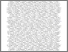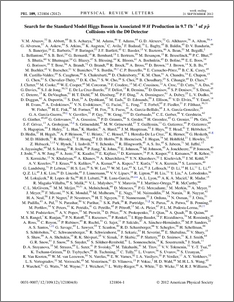# Search for the standard model Higgs boson in associated $WH$ production in 9.7 fb$^{-1}$ of $p\bar{p}$ collisions with the D0 detector

D0 Collaboration, The and Bertram, Iain and Borissov, Guennadi and Fox, Harald and Ross, Anthony and Williams, Mark and Ratoff, Peter (2012) Search for the standard model Higgs boson in associated $WH$ production in 9.7 fb$^{-1}$ of $p\bar{p}$ collisions with the D0 detector. Physical review letters, 109 (12). ISSN 1079-7114Preview
PDF
PhysRevLett.109.121804.pdf - Published Version

## Abstract

We present a search for the standard model Higgs boson in final states with a charged lepton (electron or muon), missing transverse energy, and two or three jets, at least one of which is identified as a $b$-quark jet. The search is primarily sensitive to $WH\to\ell\nu b\bar{b}$ production and uses data corresponding to 9.7 fb$^{-1}$ of integrated luminosity collected with the D0 detector at the Fermilab Tevatron $p\bar{p}$ Collider at $\sqrt{s}=1.96$ TeV. We observe agreement between data and the expected background. For a Higgs boson mass of 125 GeV, we set a 95% C.L. upper limit on the production of a standard model Higgs boson of 5.2$\times\sigma_{\rm SM}$, where $\sigma_{\rm SM}$ is the standard model Higgs boson production cross section, while the expected limit is 4.7$\times\sigma_{\rm SM}$.

Item Type:
Journal Article
Journal or Publication Title:
Physical review letters
© 2012 American Physical Society 8 pages, 3 figures, published in PRL
Uncontrolled Keywords:
/dk/atira/pure/subjectarea/asjc/3100
Subjects:
Departments:
ID Code:
63426
Deposited By:
Deposited On:
19 Apr 2013 08:38
Refereed?:
Yes
Published?:
Published